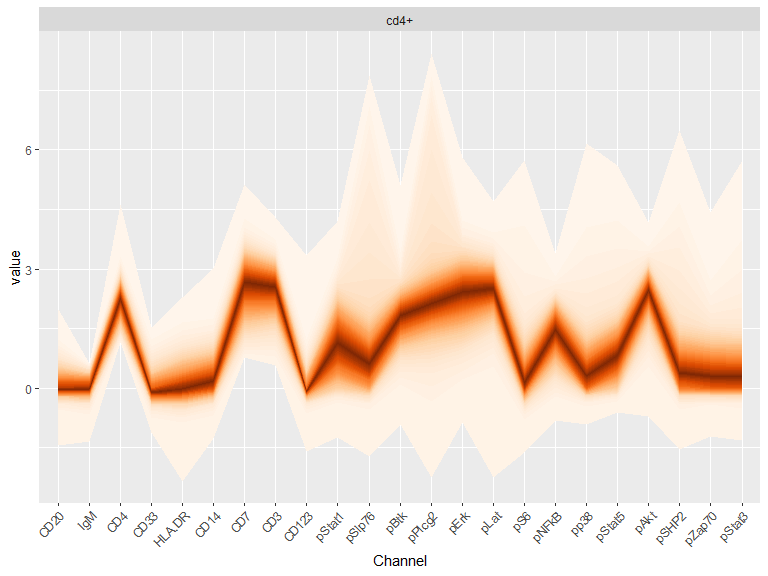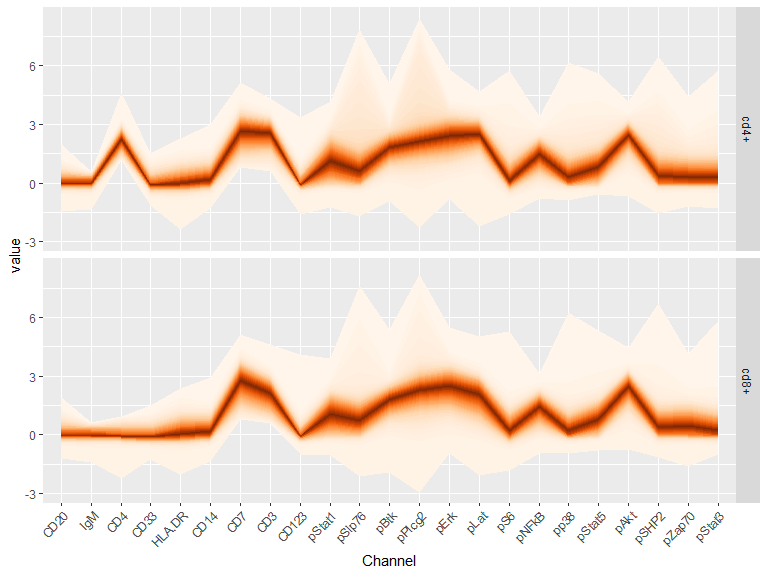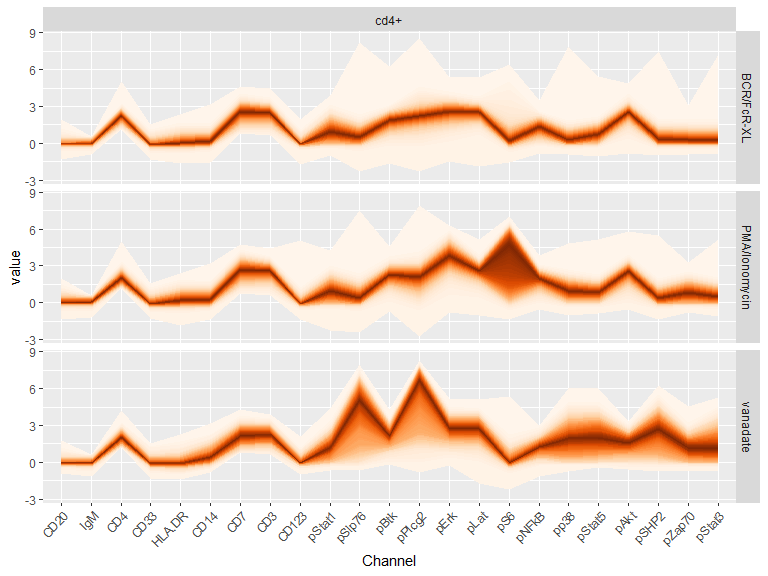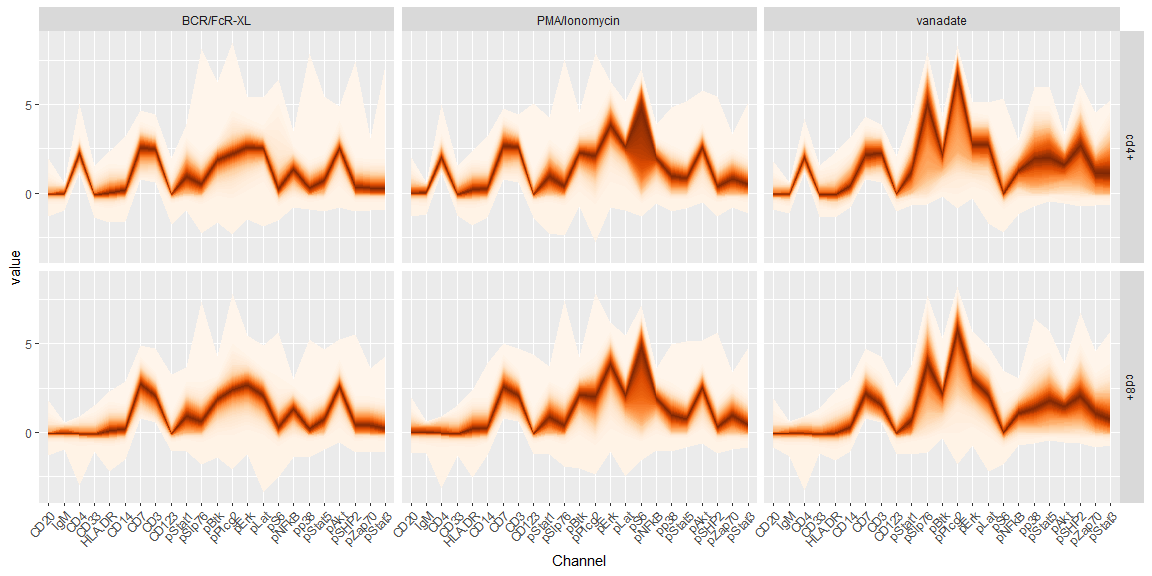# Abstract

Fan plots were originaly developed by the Bank of England to visualize trends in time series (Britton, E.; Fisher, P. & J. Whitley (1998) The Inflation Report Projections: Understanding the Fan Chart). They summarize data and provide a clear visualization of both the main trend and the uncertainty about the trend. When applied to flow or mass cytometry data, they enable the visualization of the main profile of the population under consideration, and capture at the same time the homogeneity of the population.

# Introduction

A key challenge in the analysis of high-dimensional data is visualization. In the context of population analysis, once a group of cells has been identified either through manual gating or clustering how do we efficiently check the main trend and homogeneity of this group. Fan plots are an attractive method that combines the easy interpretation of line charts, combined with an overview of the underlying distribution similar to that provided through smooth scatter plots. We will illustrate these 2 properties using an example flow cytometry dataset.

## Building a Fan plot

To build a fan plot, one first breaks the data distribution into a predefined number of bins and records the bin limits. In the default implementation (using 100 bins), the center bin corresponds to the distribution median. Each bin is plotted as a small rectangle, filled with a color shade that corresponds to the bin position. The center bin gets the strongest shade of the base color, and the color is increasingly decreased for bins that correspond to higher or smaller values. When multiple channels are plotted side-by-side, the rectangle can be joined leading to a visual metaphor similar to a line chart.

## Installation

The package can be installed using the following command:

devtools::install_github('yannabraham/cytofan')

Once installed the package can be loaded using the standard library command.

library(cytofan)
#> Loading required package: ggplot2

# Visualizing mass cytometry data from the bodenmiller package

To illustrate the use of cytofan in the context of cytometry, we will use data published in Bodenmiller et al Nat Biotech 2012. Samples corresponding to untreated cells, stimulated with BCR/FcR-XL, PMA/Ionomycin or vanadate or unstimulated, were downloaded from CytoBank as FCS files. Data was extracted and normalized using the arcsinh function with a cofactor of 5.

We assemble a dataset containing all reference samples, and both phenotypic and functional channels:

data('refPhenoMat')
data('refFuncMat')
data('refAnnots')
refFullFrame <- melt(cbind(refPhenoMat,
refFuncMat))
names(refFullFrame) <- c('cell_id','Channel','value')
refFullFrame$Channel <- factor(refFullFrame$Channel,
levels=c(colnames(refPhenoMat),
colnames(refFuncMat)))
refFullFrame$Cells <- rep(refAnnots$Cells,
ncol(refPhenoMat)+ncol(refFuncMat))

We first visualize the profile of CD4+ T cells using fan plots:

refFullFrame %>%
filter(Cells=='cd4+') %>%
ggplot(aes(x=Channel,y=value))+
geom_fan()+
facet_grid(.~Cells)+
theme(axis.text.x = element_text(angle = 45, hjust = 1))Fan plots can be used to compare populations, eg CD4+ and CD8+ T cells:

refFullFrame %>%
filter(Cells %in% c('cd4+','cd8+')) %>%
ggplot(aes(x=Channel,y=value))+
geom_fan()+
facet_grid(Cells~.)+
theme(axis.text.x = element_text(angle = 45, hjust = 1))# Visualizing functional differences after stimulation.

We assemble a dataset containing all untreated samples, stimulated with BCR/FcR-XL, PMA/Ionomycin or vanadate, and both phenotypic and functional channels:

data('untreatedPhenoMat')
data('untreatedFuncMat')
data('untreatedAnnots')
untreatedFullFrame <- melt(cbind(untreatedPhenoMat,
untreatedFuncMat))
names(untreatedFullFrame) <- c('cell_id','Channel','value')
untreatedFullFrame$Channel <- factor(untreatedFullFrame$Channel,
levels=c(colnames(untreatedPhenoMat),
colnames(untreatedFuncMat)))
untreatedFullFrame$Cells <- rep(untreatedAnnots$Cells,
ncol(untreatedPhenoMat)+ncol(untreatedFuncMat))
untreatedFullFrame$Treatment <- rep(untreatedAnnots$Treatment,
ncol(untreatedPhenoMat)+ncol(untreatedFuncMat))

We then visualize the effects of stimulation on the profile of CD4+ T cells:

untreatedFullFrame %>%
filter(Cells=='cd4+') %>%
ggplot(aes(x=Channel,y=value))+
geom_fan()+
facet_grid(Treatment~Cells)+
theme(axis.text.x = element_text(angle = 45, hjust = 1))We can also compare the effects of treatments across populations, eg CD4+ and CD8+ T cells:

untreatedFullFrame %>%
filter(Cells %in% c('cd4+','cd8+')) %>%
ggplot(aes(x=Channel,y=value))+
geom_fan()+
facet_grid(Cells~Treatment)+
theme(axis.text.x = element_text(angle = 45, hjust = 1))# Conclusion

Fan plots provide a straightforward method to compare profiles across conditions, while accounting for homogeneity of response or composition of a given compartment. Fan plots enable quick exploration and comparison of groups of cells such as manual gates and clusters.